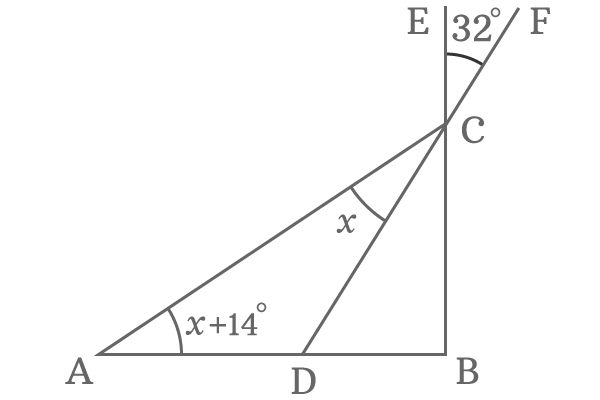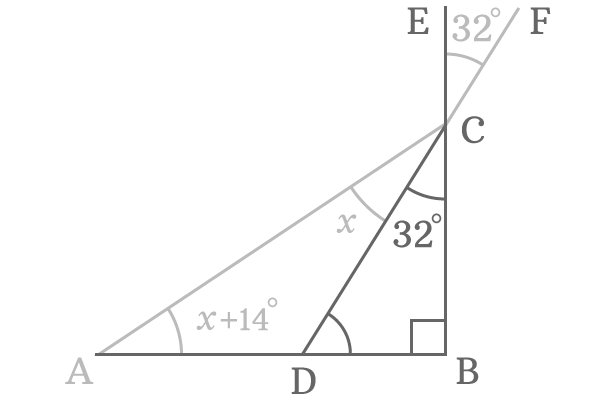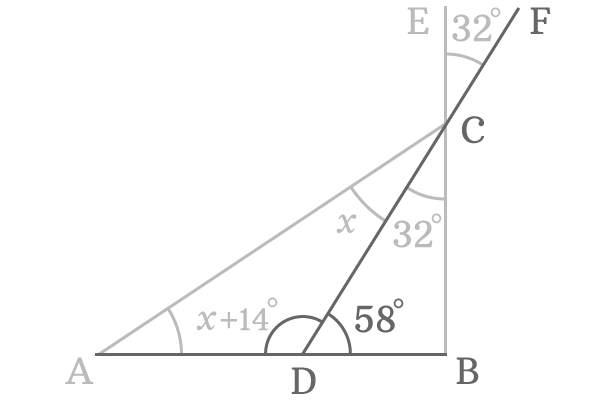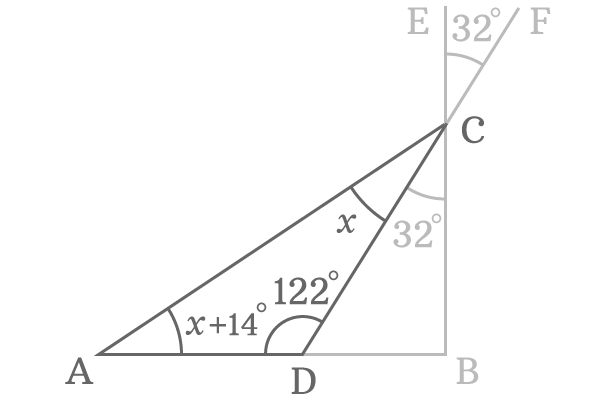# Find the value of $x$, if $AB ⊥ BE$ and $\angle ECF = 32°$$\Delta BAC$ is a right angled triangle and it is divided by the line $\overline{DF}$ as two sub triangles. The side $\overline{BC}$ is extended through $E$ to form an exterior angle $\angle ECF$ and it is measured as $32^\circ$. The sides $\overline{AB}$ and $\overline{BE}$ are perpendicular lines.

The angle of the triangle $DCA$ is $x$ and the angle of the right angled triangle $BAC$ is $x+14^\circ$ but the value of $x$ is unknown. The value of $x$ has to be evaluated by using the given data.

###### Step: 1The $\angle ECF = 32^\circ$ but $\angle BCD$ is unknown.

Geometrically, the angles $ECF$ and $BCD$ are vertically opposite angles. So, the angle $BCD$ is exactly equal to the angle $ECF$.

$\therefore \,\,\,\,\,\, \angle BCD = \angle ECF = 32^\circ$

###### Step: 2It is given in the problem that the side $\overline{AB}$ is perpendicular to the side $\overline{BE}$. So, the angle between them is $90^\circ$. The sides $\overline{DB}$ and $\overline{BC}$ both are part of the sides $\overline{AB}$ and $\overline{BE}$ respectively. Hence, the angle $\angle DBC$ is also a right angle.

$\angle DBC = 90^\circ$

The angles $DBC$ and $BCD$ are known in the right angled triangle $BDC$. So, the third triangle can be determined by using the sum of angles of the triangle rule.

$\angle BDC + \angle DBC + \angle BCD = 180^\circ$

$\implies \angle BDC + 90^\circ + 32^\circ = 180^\circ$

$\implies \angle BDC + 122^\circ = 180^\circ$

$\implies \angle BDC = 180^\circ -122^\circ$

$\therefore \,\,\,\,\,\,\,\, \angle BDC = 58^\circ$

###### Step: 3The side $\overline{AB}$ is a straight line. So, its angle $ADB$ is $180^\circ$ but the angle is divided by the line $\overline{DF}$ into two sub angles $ADF$ and $BDF$. The sum of them is equal to the $\angle ADB$ geometrically.

$\angle ADF + \angle BDF = \angle ADB$

$\implies ADF + 58^\circ = 180^\circ$

$\implies ADF = 180^\circ – 58^\circ$

$\therefore \,\,\,\,\,\,\,\, ADF = 122^\circ$

###### Step: 4

Geometrically, the $\angle ADF$ is equal to the $\angle ADC$. Now, apply the sum of angles of the triangle rule once again to obtain the value of $x$ in degrees.$\angle DAC + \angle ACD + \angle CDA = 180^\circ$

$\implies x+14^\circ + x + 122^\circ = 180^\circ$

$\implies x+x+ 122^\circ + 14^\circ = 180^\circ$

$\implies 2x + 136^\circ = 180^\circ$

$\implies 2x = 180^\circ – 136^\circ$

$\implies 2x = 44^\circ$

$\implies x = \dfrac{44^\circ}{2}$

$\require{cancel} \implies x = \dfrac{\cancel{44^\circ}}{\cancel{2}}$

$\therefore \,\,\,\,\,\,\,\, x = 22^\circ$

Therefore, the value of $x$ is $22$ degrees and it is the required solution for this geometry maths problem.

Latest Math Topics
Latest Math Problems

A best free mathematics education website for students, teachers and researchers.

###### Maths Topics

Learn each topic of the mathematics easily with understandable proofs and visual animation graphics.

###### Maths Problems

Learn how to solve the maths problems in different methods with understandable steps.

Learn solutions

###### Subscribe us

You can get the latest updates from us by following to our official page of Math Doubts in one of your favourite social media sites.